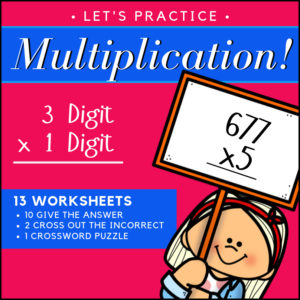Showing all 14 results

•\$3.00

Math Skills Practice Workbook has been designed to review and practice 4th and 5th grade skills. Includes 186 problems, 10 student worksheets + answer keys!

See description below for the list of included skills!

• Sale!\$6.00 \$5.00

This resource will allow students to practice converting Roman numerals as well as comparing Roman numerals to Arabic numbers! There are 20 student worksheets with 240 total questions.

Use this bundle if you need worksheets for differing levels of knowledge:

• Roman Numerals 1 to 99 (I, V, X, L)
• Roman Numerals 1 – 1600 (I, V, X, L, C, D, M)

For a fun, holiday themed twist, each worksheet as a Thanksgiving themed maze!

This product contains two MTL resources:

•\$3.00

This resource is designed to give students the practice the need to become proficient at multiplying decimals. There are three levels, 15 worksheets (16 problems each) and answer keys. That’s 240 problems – EACH WORKSHEET includes a different growth mindset quote!
(See description below for more details)

•\$3.00

This resource is designed to give students the practice the need to become proficient at multiplying decimal numbers. There are three levels, 15 worksheets (20 problems each) and answer keys. That’s 300 problems! Use as practice sheets, homework, or for quizzes and tests.

Also included: Visual poster to help students with ‘where to place’ the decimal point in their answers.

3 Levels:

• Level 1 (5 worksheets)
Tenths multiplied by tenths
• Level 2 (5 worksheets)
Tenths multiplied by hundreds and/or by thousandths
Hundredths multiplied by hundredths and/or by thousandths
• Level 3 (5 worksheets)
Mixed from tenths/hundredths/thousandths multiplied by tenths/hundredths/thousandths
•\$2.25
•\$3.25

This resource includes 27 ready to use Math worksheets for 4th-5th grade students. Designed to give students plenty of practice and to reinforce student knowledge of decimal notation, location on a number line, digit value and relative value in relation to half.

•\$2.25

These 300 problems will give your students the practice they need to reinforce their multiplication skills (single digit number x double digit)! This resource includes 10 worksheets with 30 problems each. Each can be used by students for practice or for assessment (quiz and test). Answer keys provided.

Skill: Students will multiply a two digit whole number by a one-digit whole number.

•\$4.99

OVER 1,800 problems, your students will have the practice they need to understand and learn their multiplication facts 0 to 10!

The resource begins with repeated addition (to reinforce the concept of addition in relation to multiplication) and ends with students completing all multiplication facts.

•\$2.50

Students will have a great deal of practice solving division word problems as this resource includes 96 problems designed for 4th-5th grades.

•\$3.00

Help your students reinforce their knowledge and Math skills with these 15 worksheets (210 problems) on dividing fractions! Students will divide fractions (numerator of 1) by whole numbers and whole numbers by fractions (numerator of 1).

•\$2.50

Give students the practice they need to master long division with these 15 worksheets (165 problems) resource. Students will solve division problems with 2-3 digit dividends and 1 digit divisors. (with and without remainders) – Answer Keys provided

•\$2.25

These 300 problems will give your students the practice they need to reinforce their multiplication skills (double digit x double digit)! This resource includes 15 worksheets with 20 problems each. Each can be used by students for practice or for assessment (quiz and test). Answer keys provided.

Skill: Students will multiply a double digit whole number by a double-digit whole number.

•\$2.50

This resource includes 10 worksheets (each with 12 problems). Students will be asked to identify factors and multiples of given numbers.

•\$3.00

These 500 problems will give your students the practice they need to reinforce their multiplication skills (triple digit x single – double – triple digit)! Can be used by students for practice or for assessment (quiz and test). Answer keys provided.

Skill: Students will multiply a triple digit whole number by a single, double or triple digit whole number.

Includes:

• – 5 Worksheets (triple x single digit) 125 problems total
• – 5 Worksheets (triple x double digit) 100 problems total
• – 5 Worksheets (triple x triple digit) 75 problems total
• – 5 Worksheets (mixed – triple x single, double and triple digit) 75 problems total